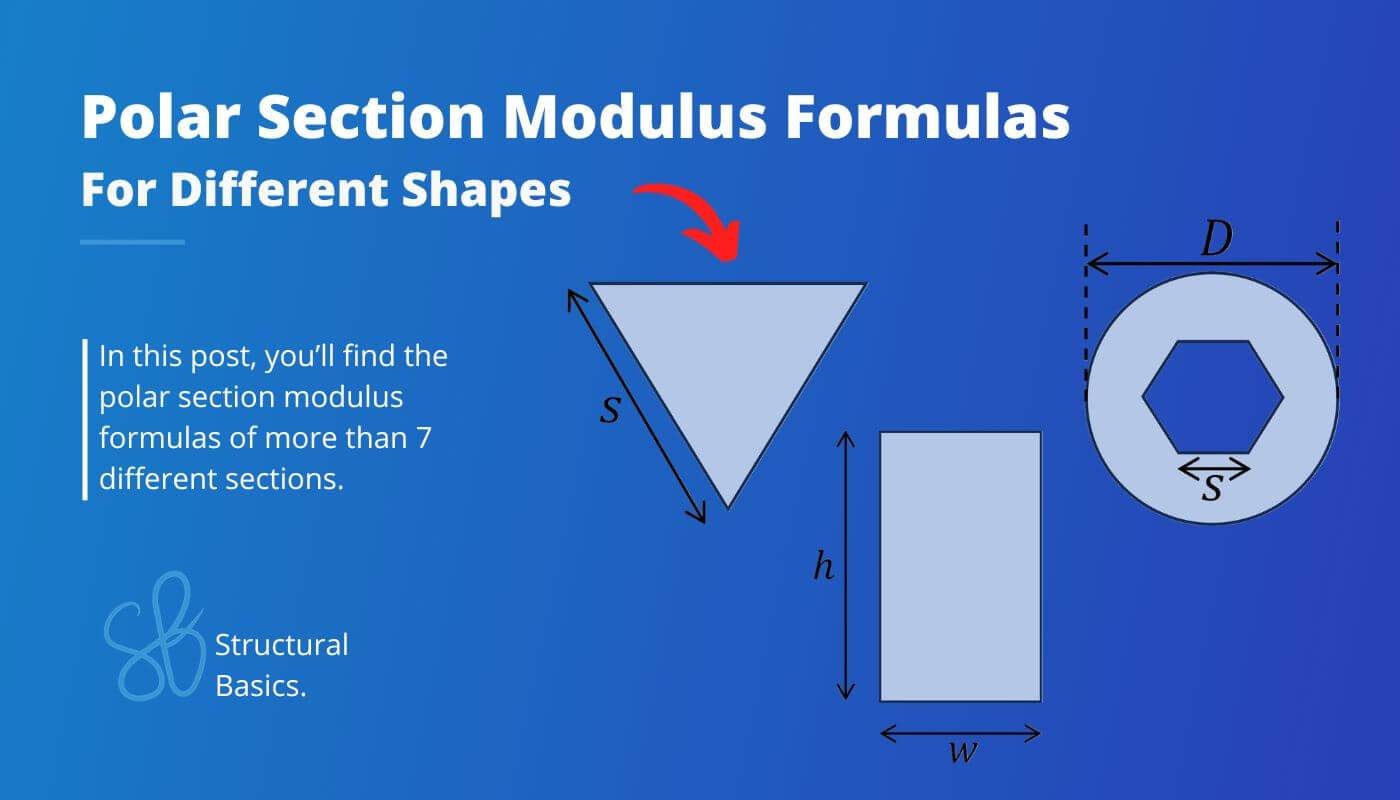# 7+ Polar Section Modulus FormulasThe polar moment of inertia, quantifies an object/section’s resistance to rotational motion about a specific axis.

This property plays an important role in structural and mechanical engineering, ranging from bolted steel connections to complex machinery analysis.

Every shape has a different polar moment of inertia. In this blog post, we’ll show the formulas for different sections, so you don’t have to calculate them yourself.

Let’s get started. 🚀🚀

## What Is The Polar Section Modulus?

The polar section modulus, often denoted as Wp or Z, is a measure of the resistance of a structural shape (cross-section) to torsional/twisting loads.

It is commonly used in engineering and mechanics to analyse and design structures, components and connections subjected to torsional loads, such as shafts, steel tubes and bolted connections.

## Formula Of Polar Section Modulus

The formula for the polar section modulus is the following:

$$W_p = \frac{I_p}{r}$$

With,

Check out this article to learn how to calculate the polar moment of inertia.

## 1. Square Section

Polar section modulus

$W_p = 0.074 w^3$

### Example calculation

w = 120 mm

$W_p = 0.074 w^3 = 0.074 \cdot (120mm)^3 = 1.28 \cdot 10^5 mm^3$

## 2. Rectangular Section

Polar section modulus

$W_p = \frac{h \cdot w^2}{3 + 1.8 \cdot \frac{w}{h}}$

### Example calculation

w = 120 mm, h = 240mm

$W_p = \frac{h \cdot w^2}{3 + 1.8 \cdot \frac{w}{h}} = \frac{240mm \cdot (120mm)^2}{3 + 1.8 \cdot \frac{120mm}{240mm}} = 8.86 \cdot 10^5 mm^3$

## 3. Circle/ Circular Section

Polar section modulus

$W_p = \frac{\pi \dot D^3}{16}$

### Example calculation

D = 200 mm

$W_p = \frac{\pi \dot D^3}{16} = \frac{\pi \dot (200mm)^3}{16} = 1.57 \cdot 10^6 mm^3$

## 4. Circular Tube Section

Polar section modulus

$W_p = \frac{\pi}{16} \cdot (\frac{D^4 – d^4}{D})$

### Example calculation

D = 200 mm, d = 180mm

$W_p = \frac{\pi}{16} \cdot (\frac{D^4 – d^4}{D}) = \frac{\pi}{16} \cdot (\frac{(200mm)^4 – (180mm)^4}{200mm}) = 5.4 \cdot 10^5 mm^3$

## 5. Circular Section With Square Cutout

Polar section modulus

$W_p = \frac{\pi \cdot D^3}{16} – \frac{s^4}{3D}$

### Example calculation

D = 200 mm, s = 100 mm

$W_p = \frac{\pi \cdot D^3}{16} – \frac{s^4}{3D} = \frac{\pi \cdot (200mm)^3}{16} – \frac{(100mm)^4}{3 \cdot 200mm} = 5.2 \cdot 10^6 mm^3$

## 6. Hexagon Section

Polar section modulus

$W_p = 0.2 s^3$

### Example calculation

s = 200 mm

$W_p = 0.2 s^3 = 0.2 (200mm)^3 = 1.6 \cdot 10^6 mm^3$

## 7. Triangular Section

Polar section modulus

$W_p = \frac{s^3}{20}$

### Example calculation

s = 100 mm

$W_p = \frac{s^3}{20} = \frac{s^3}{20} = 5.0 \cdot 10^4 mm^3$

## 8. Circular Section With Socket Head Cutout

Polar section modulus

$W_p = \frac{\pi D^3}{16} – \frac{5 \sqrt{3}}{4D} \cdot s^4$

### Example calculation

D = 300 mm, s = 100 mm

$W_p = \frac{\pi D^3}{16} – \frac{5 \sqrt{3}}{4D} \cdot s^4$

$W_p = \frac{\pi (300mm)^3}{16} – \frac{5 \sqrt{3}}{4 \cdot 300mm} \cdot (100mm)^4$

$W_p = 4.6 \cdot 10^6 mm^3$

## Conclusion

The polar section modulus is an important parameter in structural design of a few elements. Therefore, it’s recommendable to know how to calculate it for different cross-sections.

In the following blog posts, we show step-by-step, how to calculate moments of inertias.

If you are new to structural design, then check out our design tutorials about other structural elements. 👇👇

Do you miss any 2nd polar moment of inertia formula for any shape or cross-section that we forgot in this article? Let us know in the comments. ✍️✍️